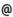## "Babes-Bolyai" University of Cluj-Napoca Faculty of Mathematics and Computer Science

 Computational mechanics
 Code Semes-ter Hours: C+S+L Credits Type Section MM275 1 2+2+0 9 compulsory Matematică Computaţională - în limba maghiară
 Teaching Staff in Charge
 Assoc.Prof. SZENKOVITS Ferenc, Ph.D., fszenkomath.ubbcluj.ro
 Aims The aim of this course is to give a short introduction in modeling of dynamical systems with a finite number of degrees. Students absolving this course will be able to study dynamical problems using Lagrangian end Hamiltonian formalism. They will be able to study various phenomenon using numerical methods.
 Content I. Mathematical methods for differential equations 1. Models and differential equations 2. Models and mathematical problems 3. Stability and perturbation methods II. Mathematical methods of classical mechanics 1. Dynamical systems and Newtonian dynamics 2. Lagrangian dynamics 3. Hamiltonian dynamics and Hamilton-Jacobi Theory III. Chaotic dynamics, stability and bifurcations 1. Stability diagrams 2. Limit cycles 3. Hopf bifurcation 4. Chaotic motions IV. Numerical methods for ordinary differential equations 1. Numerical methods for initial-value problems 2. Numerical methods for boundary-value problems V. Applications
 References 1. ARNOLD, V.I.: Metodele matematice ale mecanicii clasice, Editura Stiintifica si Enciclopedica, Bucuresti, 1980. 2. BELLAMO, N., PREZIOSI, L., ROMANO, A.: Mechanics and Dynamical Systems with Mathematica, Birkhauser, 2000. 3. BUTCHER, J. C.: The numerical analysis of ordinary differential equations. John Wiley Sons, 1987. 4. DRAGOS, L.: Principiile mecanicii analitice, Editura Tehnica, Bucuresti, 1976. 5. BÁLINT, ÉRDI: The dynamics of the Solar System, ELTE Eötvöos Kiadó, Budapest, 2001 (Hungarian). 6. NAKAMURA, S.: Numerical analysis and graphic visualization with Matlab, Prestice Hall PTR, New-Jersey, 1996. 7. PRESS, W. H., TEUKOLSKY, S. A., VETTERLING, W. T., FLANNERY, B. P.: Numerical Recipes in C, The Art of Scientific Computing, Second Edition, Cambridge University Press, Cambridge, New York, Port-Chester, Melbourne, Sydney, 1992. 8. SZENKOVITS F., MAKO Z., CSILLIK I., BÁLINT, A.: Mechanikai rendszerek számítógépes modellezése, Ed. Sapientia, Cluj-Napoca, 2002.
 Assessment Project - 50% and a practical verification - 50%.